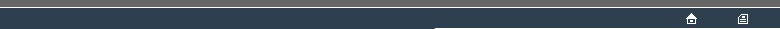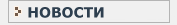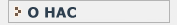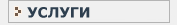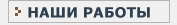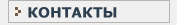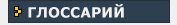СТАТИСТИЧЕСКИЙ В данном разделе Вы найдете все интересующие Вас термины и определения, связанные с статистикой и статистическими исследованиями.КЛИНИЧЕСКИЕ ИССЛЕДОВАНИЯ Необходимый перечень терминов и определений, используемых при проведении клинических испытанийO OBJECT CODE This is the code which a COMPUTER recognises and acts upon as a direct consequence of its electromechanical construction. Typically such code is highly abstract and unsuitable for use in general use by human programmers. The OBJECT CODE to specify a certain process is usually generated through use of a COMPILER. Also see : PROGRAMMING LANGUAGE. ODDS RATIO An alternative characterisation of the parameter 'p' for a BINOMIAL PROCESS is the ratio of the incidences of the two alternatives : p/(1-p) ; this quantity is termed the ODDS RATIO; the value may range from zero to infinity. This relates to a possible view of a BINOMIAL PROCESS as the combined activity of two POISSON PROCESSes with a limit upon total count for the two processes combined. Also see : LOGISITIC REGRESSION. ORDINAL SCALE A MEASUREMENT TYPE for which the relative values of data are defined solely in terms of being lesser, equa-to or greater as compared with other data on the ORDINAL SCALE. These characteristics may arise from categorical rating scales, or from converting INTERVAL SCALE data to become RANKED DATA. OUTCOME VALUE The value of the TEST STATISTIC for the data as initially observed, before any RE-RANDOMISATION..O
OBJECT CODE
ODDS RATIO
ORDINAL SCALE
OUTCOME VALUEНовости   О нас   Услуги   Наши работы   Статьи   Контакты   Глоссарий
Статистическая помощь! © 2005 - 2022 • Защита авторских прав